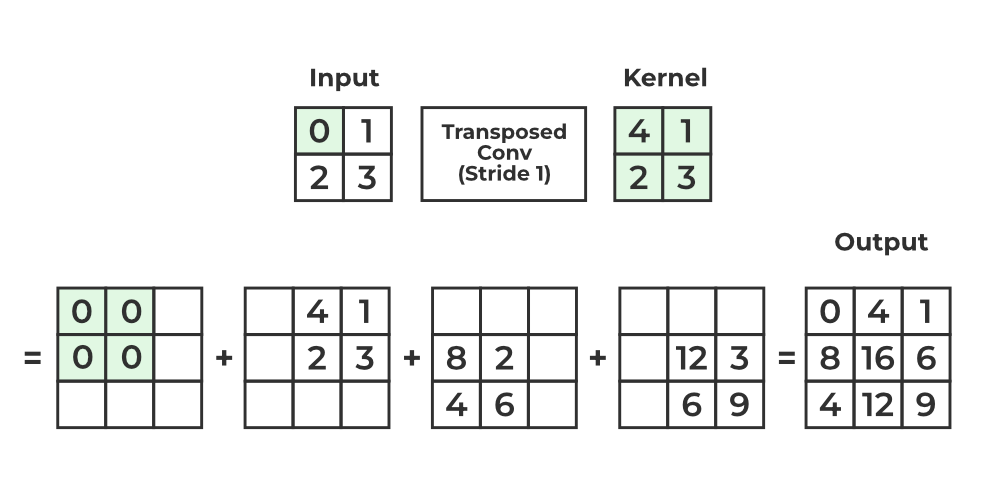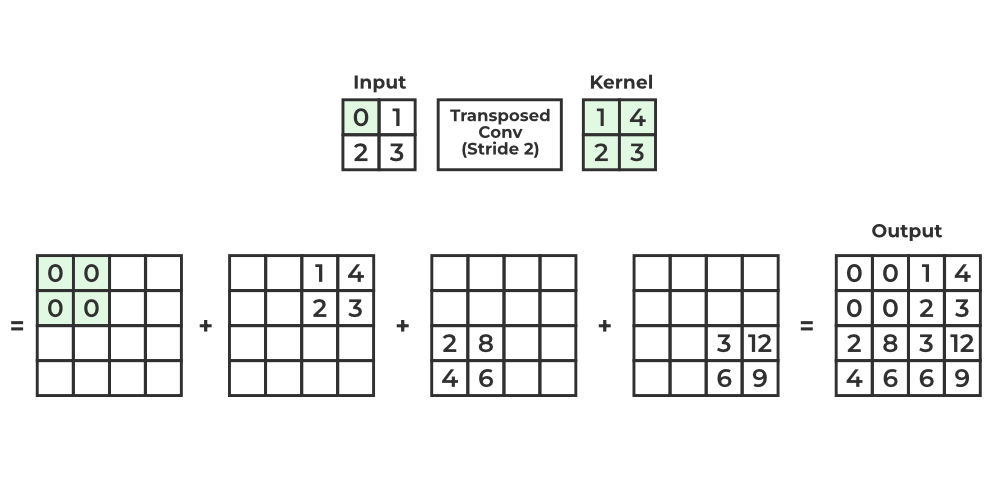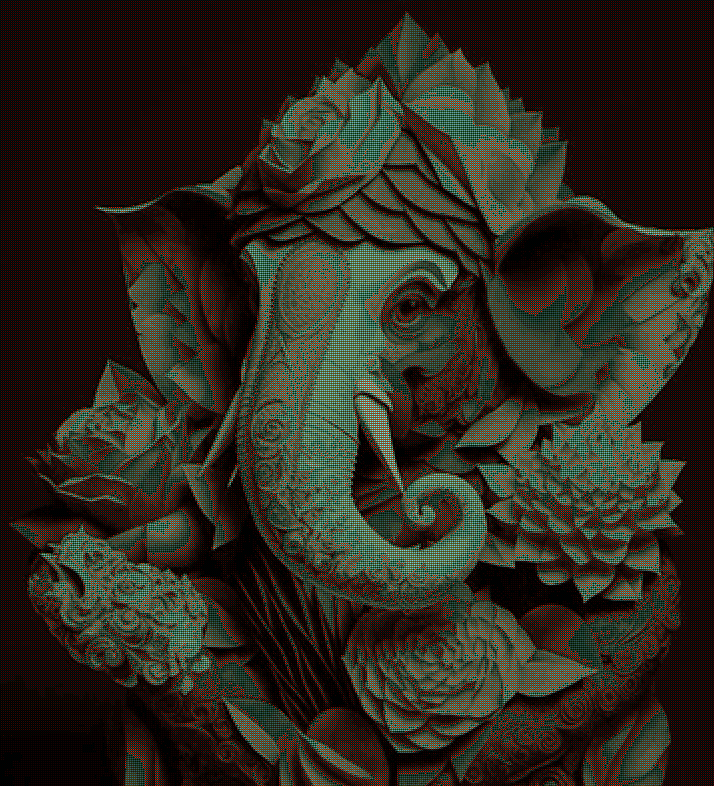Open in App
Not now

# Apply a 2D Transposed Convolution Operation in PyTorch

• Last Updated : 22 Feb, 2023

Transposed convolution, also known as fractionally-strided convolution, is a technique used in convolutional neural networks (CNNs) for the upsampling layer that increases the spatial resolution of an image. It is similar to a deconvolutional layer. A deconvolutional layer reverses the layer to a standard convolutional layer. If the output of the standard convolution layer is deconvolved with the deconvolutional layer then the output will be the same as the original value, While in transposed convolutional value will not be the same, it can reverse to the same dimension,Transposed Convolutional  Stride = 1

In this article, we will discuss how to apply a 2D transposed convolution operation in PyTorch.

Before diving into the implementation of transposed convolution in PyTorch, let’s first understand the basic concepts related to the topic.

• Convolution: Convolution is a mathematical operation that applies a filter to an image to extract features. In CNNs, a convolutional layer applies a set of filters to an input image to extract features.
• Transposed Convolution: Transposed convolution, also known as fractionally-strided convolution or deconvolution, is the reverse operation of convolution. It is used to increase the spatial resolution of an image by expanding the feature maps produced by a convolutional layer.
• Stride: Stride is the number of pixels by which the filter moves in the image. A larger stride means fewer feature maps, while a smaller stride results in more feature maps.
• Padding: Padding is the number of pixels added to the edges of an image to preserve its spatial size after convolution.
• Output Shape: The output shape of a transposed convolution operation depends on the input shape, the kernel size, the stride, and the padding.

In a transposed convolutional layer, the input is a feature map of size, whereandare the height and width of the input and the kernel size is, whereandare the height and width of the kernel.

If the stride shape isand padding is p, The stride of the transposed convolutional layer determines the step size for the input indices p and q, and the padding determines the number of pixels to add to the edges of the input before performing the convolution. Then the output of the transposed convolutional layer will bewhereandare the height and width of the output.

### Example 1:

Suppose we have a grayscale image of size 2 X 2, and we want to upsample it using a transposed convolutional layer with a kernel size of 2 x 2, a stride of 2, and zero padding (or no padding). The input image and the kernel for the transposed convolutional layer would be as follows:The output will be:Transposed Convolutional  with stride 2

Code Explanations:

• Import necessary libraries (torch and nn from torch)
• Define Input tensor and custom kernel
• Redefine the shape in 4 dimensions because PyTorch takes 4D shapes in inputs.
• Apply Transpose convolution with input and output channel =1,1, kernel size =2, stride = 2, padding = 0 means valid padding.
• Set the customer kernel weight by using Transpose.weight.data
• Apply Transpose convolution on input data.

## Python3

 # Import the necessary moduleimport torchfrom torch import nn # InputInput = torch.tensor([[0.0, 1.0], [2.0, 3.0]])#KernelKernel = torch.tensor([[4.0, 1.0], [2.0, 3.0]]) # Redefine the shape in 4 dimensionInput = Input.reshape(1, 1, 2, 2)Kernel = Kernel.reshape(1, 1, 2, 2) # Transpose convolution LayerTranspose = nn.ConvTranspose2d(in_channels =1,                               out_channels =1,                               kernel_size=2,                               stride = 2,                               padding=0,                               bias=False) # Initialize KernelTranspose.weight.data = Kernel# Output valueTranspose(Input)

Output:

tensor([[[[ 0.,  0.,  4.,  1.],
[ 0.,  0.,  2.,  3.],
[ 8.,  2., 12.,  3.],
[ 4.,  6.,  6.,  9.]]]], grad_fn=<ConvolutionBackward0>)

The output shape can be calculated as :### Example:2

Let’s create a tensor of shape (1,1,4,4) with torch.randn() and apply transpose convolution with torch.nn.ConvTranspose2d with pytorch with kernel size(3,3) and stride(2,2) and padding (1,1).

## Python

 import torchimport torch.nn as nn # Define input imageinput_image = torch.randn(1, 1, 4, 4)print('Input Shape:',input_image.shape)# Define kernel sizekernel_size = (3, 3) # Define stridestride = (2, 2) # Define paddingpadding = (1, 1) # Define transposed convolution layertransposed_conv = nn.ConvTranspose2d(in_channels=1,                                     out_channels=1,                                     kernel_size=kernel_size,                                     stride=stride,                                     padding=padding) # Perform transposed convolutionoutput = transposed_conv(input_image) # Display outputprint("output \n", output)print("\n output Shape", output.shape)

Output:

Input Shape: torch.Size([1, 1, 4, 4])
output
tensor([[[[ 0.2094,  0.3711,  0.1221,  0.0517,  0.4600,  0.0966,  0.4605],
[ 0.1893,  0.2858,  0.2451,  1.0030,  0.7390, -0.6206, -0.0103],
[ 0.1951,  0.2099,  0.2970, -0.1894,  0.7507,  0.6869, -0.1451],
[-0.2257,  0.8582,  0.3090,  0.5730,  0.4639,  0.2012,  0.2094],
[-0.2951,  0.1390,  0.3026,  0.2176,  0.3044,  0.1649,  0.3625],
[ 0.3149,  0.3095,  0.5061, -0.0233,  0.2429,  0.6422,  0.4626],
[ 0.5492,  0.0399,  0.5359,  0.3251,  0.2207,  0.0652,  0.4598]]]],

output Shape torch.Size([1, 1, 7, 7])

The output shape can be calculated as :### Example 3:

Let’s apply the transpose convolution on a real image here we will read the image with PIL library PIL.image() function and convert it into PyTorch tensor with torchvision.transforms.ToTensor() and then applying custom kernel. if here we will not define the kernel the output will refresh every time on running the because of random kernel. here kernel size is 2, stride = 2 and padding = 1.

Input ImageInput Image

## Python

 # Import the necessary modulefrom PIL import Imageimport torchfrom torch import nnfrom torchvision import transforms # Read input imageimg = Image.open('Ganesh.jpg') # convert the input image to torch tensorimg = transforms.ToTensor()(img)print("Input image size:", img.size()) # unsqueeze the image to make it 4D tensorimg = img.unsqueeze(0)print('unsqueeze Image size',img.shape) #KernelKernel = torch.tensor([    [[[1.0,  0.1],[ 0.1, 0.2]],[[ 0.1, 0.2],[ 0.2,  0.3]],[[ 0, 0.1],[0.2, 0.3]]],    [[[1.0,  0.1],[ 0.1, 0.2]],[[ 0.1, 0.2],[ 0.2,  0.3]],[[ 0, 0.1],[0.2, 0.3]]],    [[[1.0,  0.1],[ 0.1, 0.2]],[[ 0.1, 0.2],[ 0.2,  0.3]],[[ 0, 0.1],[0.2, 0.3]]],]) # Kernel shapeprint('Kernel Size :',Kernel.shape)  # Transpose convolution LayerTranspose = nn.ConvTranspose2d(in_channels =3,                               out_channels =2,                               kernel_size=2,                               stride = 2,                               padding=1,                               bias=False) # Initialize KernelTranspose.weight.data = Kernel # Output valueimg2 = Transpose(img) # squeeze image to make it 3Dimg2 = img2.squeeze(0)print("Output image size:",img2.size()) # convert image to PIL imageimg2 = transforms.ToPILImage()(img2) # display the image after convolutionimg2

Output:

Input image size: torch.Size([3, 394, 358])
unsqueeze Image size torch.Size([1, 3, 394, 358])
Kernel Size : torch.Size([3, 3, 2, 2])
Output image size: torch.Size([3, 786, 714])Output Image

The output shape can also be calculated as :My Personal Notes arrow_drop_up